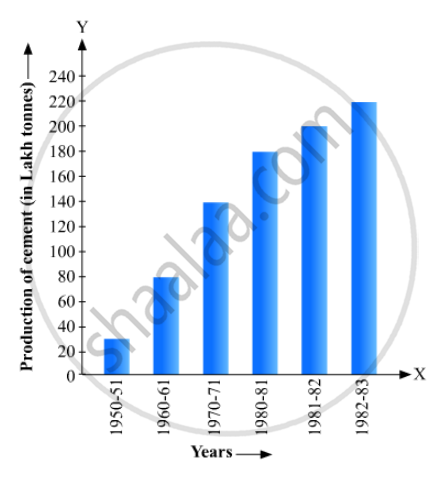# Read the Following Bar Graph(Fig. 23.15)And Answer the Following Questions: (I) What Information is Given by the Bar Graph? (Ii) What Was the Production of a Student in the Year 1980 - 81? - Mathematics

(i) What information is given by the bar graph?
(ii) What was the production of a student in the year 1980 - 81?
(iii) What is the minimum and maximum productions of cement and corresponding years?#### Solution

i) It gives information regarding industrial production of cement in different years in India.
(ii) The production of cement in the year 1980 – 1981 = 186 lakh tones.
(iii) The minimum production is 30 lakh tones in 1950 – 1951 and maximum production 232 lakh tones in 1982 – 1983

Concept: Graphical Representation of Data
Is there an error in this question or solution?

#### APPEARS IN

RD Sharma Mathematics for Class 9
Chapter 23 Graphical Representation of Statistical Data
Exercise 23.1 | Q 11 | Page 12

Share